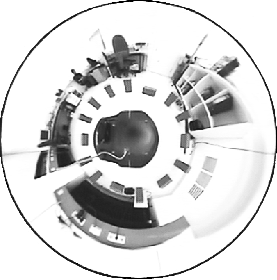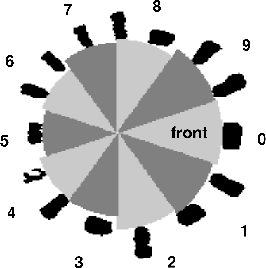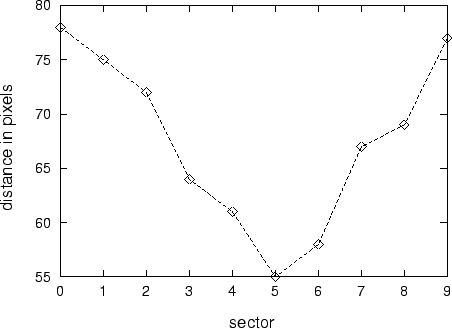Next: 7.2.4 Forward model: Multi-layer Up: 7.2 Methods Previous: 7.2.2.2 Test data

## 7.2.3 Image processing

It proved to be impossible to use the original visual information in the training for the following reasons. First, the dimensionality is too high, and second, the color of single pixels may alter drastically even for small changes in the robot's location. If each pixel value would represent a single dimension, the jumps in sensorimotor space would be too large for any function approximation. Therefore, the image was preprocessed to detect only a special class of objects and extract only a visual distance information in a few sectors. Image processing contained the following steps:

First, a contrast mechanism enhanced red objects ( R - (G + B)/2). The result was smoothened with a binomial filter. Then, a threshold function was applied on all pixel values (figure 7.3, right). Within the obtained binary image, in ten sectors (36o each), the distance from the center of the robot to the closest object was determined (figure 7.3, right). These ten distance values form the final representation of the sensory input to be processed by the network (figure 7.4). Such a sensory representation in sector values was also used by Tani (1996). Different from our model, he used a laser sensor instead of vision.

Figure 7.3: (Left) Image as seen through the mirror. (Right) Distance information in ten sectors derived from the image on the left.The `motor commands' vL and vR together with two corresponding image representations (as in figure 7.4) from two consecutive recordings (2 sec apart) make one training pattern. Each pattern is therefore a 22-dimensional vector. Before network training, the set of training patterns was normalized to have zero mean and unit variance in each dimension. The resulting normalization constants were also applied to the test set and the robot experiments.Next: 7.2.4 Forward model: Multi-layer Up: 7.2 Methods Previous: 7.2.2.2 Test data
Heiko Hoffmann
2005-03-22